# 29 Results

View
Selected filters:
• Space MathUnrestricted Use
Public Domain
Rating

This is a book containing over 200 problems spanning over 70 specific topic areas covered in a typical Algebra II course. Learners can encounter a selection of application problems featuring astronomy, earth science and space exploration, often with more than one example in a specific category. Learners will use mathematics to explore science topics related to a wide variety of NASA science and space exploration endeavors. Each problem or problem set is introduced with a brief paragraph about the underlying science, written in a simplified, non-technical jargon where possible. Problems are often presented as a multi-step or multi-part activities. This book can be found on the Space Math@NASA website.

Subject:
Mathematics
Astronomy
Material Type:
Lesson
Provider:
NASA
Provider Set:
Space Math
11/05/2014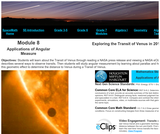Unrestricted Use
Public Domain
Rating

Students will learn about the Transit of Venus through reading a NASA press release and viewing a NASA eClips video that describes several ways to observe transits. Then students will study angular measurement by learning about parallax and how astronomers use this geometric effect to determine the distance to Venus during a Transit of Venus. This activity is part of the Space Math multimedia modules that integrate NASA press releases, NASA archival video, and mathematics problems targeted at specific math standards commonly encountered in middle school textbooks. The modules cover specific math topics at multiple levels of difficulty with real-world data and use the 5E instructional sequence.

Subject:
Mathematics
Astronomy
Material Type:
Lesson
Provider:
NASA
Provider Set:
Space Math
11/05/2014Unrestricted Use
Public Domain
Rating

This collection of activities is based on a weekly series of space science problems distributed to thousands of teachers during the 2009-2010 school year. They were intended for students looking for additional challenges in the math and physical science curriculum in grades 9 through 12. The problems were created to be authentic glimpses of modern science and engineering issues, often involving actual research data. The problems were designed to be one-pagers with a Teachers Guide and Answer Key as a second page. This compact form was deemed very popular by participating teachers.

Subject:
Mathematics
Atmospheric Science
Material Type:
Lesson
Provider:
NASA
Provider Set:
Space Math
05/02/2017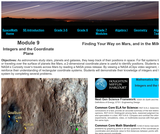Unrestricted Use
Public Domain
Rating

This is a set of three, one-page problems about how astronomers use coordinate systems. Learners will plot a constellation on a coordinate plane and/or plot the route of Mars Science Lab (MSL aka Curiosity) on the surface of Mars. Options are presented so that students may learn about the MSL mission through a NASA press release or about the coordinate plane by viewing a NASA eClips video [7 min.]. This activity is part of the Space Math multi-media modules that integrate NASA press releases, NASA archival video, and mathematics problems targeted at specific math standards commonly encountered in middle school.

Subject:
Mathematics
Astronomy
Material Type:
Lesson
Provider:
NASA
Provider Set:
Space Math
11/05/2014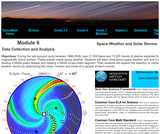Unrestricted Use
Public Domain
Rating

During the last sunspot cycle between 1996-2008, over 21,000 flares and 13,000 clouds of plasma exploded from the Sun's magnetically active surface. These events create space weather. Students will learn more about space weather and how it affects Earth through reading a NASA press release and viewing a NASA eClips video segment. Then students will explore the statistics of various types of space weather storms by determining the mean, median and mode of a sample of storm events. This activity is part of the Space Math multimedia modules that integrate NASA press releases, NASA archival video, and mathematics problems targeted at specific math standards commonly encountered in middle school textbooks. The modules cover specific math topics at multiple levels of difficulty with real-world data and use the 5E instructional sequence.

Subject:
Mathematics
Astronomy
Material Type:
Lesson
Provider:
NASA
Provider Set:
Space Math
11/05/2014Unrestricted Use
Public Domain
Rating

This book offers an introduction to the electromagnetic spectrum using examples of data from a variety of NASA missions and satellite technologies. The 84 problem sets included allow students to explore the concepts of waves, wavelength, frequency, and speed; the Doppler Shift; light; and the energy carried by photons in various bands of the spectrum. Extensive background information is provided which describes the nature of electromagnetic radiation.

Subject:
Mathematics
Astronomy
Material Type:
Lesson
Provider:
NASA
Provider Set:
Space Math
11/05/2014Unrestricted Use
Public Domain
Rating

This is an activity about the solar activity cycle. Learners will construct a graph to identify a pattern of the number of observed sunspots and the number of coronal mass ejections emitted by the Sun over a fifteen year time span. A graphing calculator is recommended, but not required, for this activity. This is the second activity in the Solar Storms and You: Exploring the Wind from the Sun educator guide.

Subject:
Mathematics
Astronomy
Material Type:
Lesson
Provider:
NASA
Provider Set:
Space Math
11/05/2014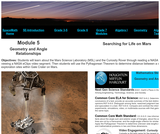Unrestricted Use
Public Domain
Rating

This is a set of four, one-page problems about the distance craft travel on Mars. Learners will use the Pythagorean Theorem to determine distance between a series of hypothetical exploration sites within Gale Crater on Mars. Options are presented so that students may learn about the Mars Science Laboratory (MSL) mission through a NASA press release or by viewing a NASA eClips video [6 min.]. This activity is part of the Space Math multi-media modules that integrate NASA press releases, NASA archival video, and mathematics problems targeted at specific math standards commonly encountered in middle school.

Subject:
Mathematics
Astronomy
Material Type:
Lesson
Provider:
NASA
Provider Set:
Space Math
11/05/2014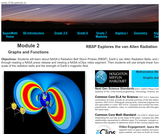Unrestricted Use
Public Domain
Rating

Students will learn about NASA's Radiation Belt Storm Probes (RBSP), Earth's van Allen Radiation Belts, and space weather through reading a NASA press release and viewing a NASA eClips video segment. Then students will use simple linear functions to examine the scale of the radiation belts and the strength of Earth's magnetic field. This activity is part of the Space Math multimedia modules that integrate NASA press releases, NASA archival video, and mathematics problems targeted at specific math standards commonly encountered in middle school textbooks. The modules cover specific math topics at multiple levels of difficulty with real-world data and use the 5E instructional sequence.

Subject:
Mathematics
Astronomy
Material Type:
Lesson
Provider:
NASA
Provider Set:
Space Math
11/05/2014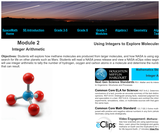Unrestricted Use
Public Domain
Rating

This is a set of three, one-page problems about the size and area of solar panels used to generate power. Learners will will use integer arithmetic to tally the number of hydrogen, oxygen and carbon atoms in a molecule and determine the number of methane atoms that can result. Options are presented so that students may learn about how NASA is using signs of methane gas to search for life on other planets, such as Mars, through a NASA press release or about how astrobiologists who are looking for life beyond Earth are using spectroscopy to identify methane plumes on Mars by viewing a NASA eClips video [7 min.]. This activity is part of the Space Math multi-media modules that integrate NASA press releases, NASA archival video, and mathematics problems targeted at specific math standards commonly encountered in middle school.

Subject:
Mathematics
Astronomy
Material Type:
Lesson
Provider:
NASA
Provider Set:
Space Math
11/05/2014Unrestricted Use
Public Domain
Rating

This is a booklet containing 37 space science mathematical problems, several of which use authentic science data. The problems involve math skills such as unit conversions, geometry, trigonometry, algebra, graph analysis, vectors, scientific notation, and many others. Learners will use mathematics to explore science topics related to Earth's magnetic field, space weather, the Sun, and other related concepts. This booklet can be found on the Space Math@NASA website.

Subject:
Mathematics
Astronomy
Material Type:
Lesson Plan
Provider:
NASA
Provider Set:
Space Math
11/05/2014Unrestricted Use
Public Domain
Rating

This book contains 24 illustrated math problem sets based on a weekly series of space science problems. Each set of problems is contained on one page. The problems were created to be authentic glimpses of modern science and engineering issues, often involving actual research data. Learners will use mathematics to explore problems that include basic scales and proportions, fractions, scientific notation, algebra, and geometry.

Subject:
Mathematics
Astronomy
Material Type:
Lesson
Provider:
NASA
Provider Set:
Space Math
11/05/2014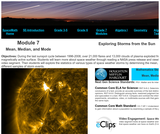Unrestricted Use
Public Domain
Rating

During the last sunspot cycle between 1996-2008, over 21,000 flares and 13,000 clouds of plasma exploded from the Sun's magnetically active surface. Students will learn more about space weather through reading a NASA press release and viewing a NASA eClips video segment. Then students will explore the statistics of various types of space weather storms by determining the mean, median and mode of different samples of storm events. This activity is part of the Space Math multimedia modules that integrate NASA press releases, NASA archival video, and mathematics problems targeted at specific math standards commonly encountered in middle school textbooks. The modules cover specific math topics at multiple levels of difficulty with real-world data and use the 5E instructional sequence.

Subject:
Mathematics
Astronomy
Material Type:
Lesson
Provider:
NASA
Provider Set:
Space Math
11/05/2014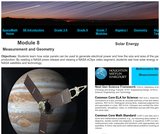Unrestricted Use
Public Domain
Rating

This is a set of three, one-page problems about the size and area of solar panels used to generate power. Learners will calculate area fractions to compare the sizes and distances of Jupiter's moons. Options are presented so that students may learn about the Juno mission through a NASA press release or about how solar energy is used by various NASA satellites and technology by viewing a NASA eClips video [3 min.]. This activity is part of the Space Math multi-media modules that integrate NASA press releases, NASA archival video, and mathematics problems targeted at specific math standards commonly encountered in middle school.

Subject:
Mathematics
Astronomy
Material Type:
Lesson
Provider:
NASA
Provider Set:
Space Math
11/05/2014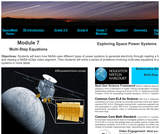Unrestricted Use
Public Domain
Rating

This is a set of three, one-page problems about mass and power of spacecraft. Learners will use multi-step equations to solve several diverse problems. Options are presented so that students may learn about different types of power systems to generate electricity through a NASA press release or by viewing a NASA eClips video [7 min.]. This activity is part of the Space Math multi-media modules that integrate NASA press releases, NASA archival video, and mathematics problems targeted at specific math standards commonly encountered in middle school.

Subject:
Mathematics
Astronomy
Material Type:
Lesson
Provider:
NASA
Provider Set:
Space Math
11/05/2014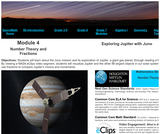Unrestricted Use
Public Domain
Rating

This is a set of two, one-page problems about the sizes of moons in the solar system. Learners will use fractions to compare the sizes and distances of Jupiter's moons. Options are presented so that students may learn about the Juno mission through a NASA press release or by viewing a NASA eClips video [6 min.] about the creation of graphic of the 88 largest objects in our solar system. This activity is part of the Space Math multi-media modules that integrate NASA press releases, NASA archival video, and mathematics problems targeted at specific math standards commonly encountered in middle school.

Subject:
Mathematics
Astronomy
Material Type:
Lesson
Provider:
NASA
Provider Set:
Space Math
11/05/2014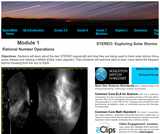Unrestricted Use
Public Domain
Rating

Students will learn about the twin STEREO spacecraft and how they are being used to track solar storms through reading a NASA press release and viewing a NASA eClips video segment. Then students will examine data to learn more about the frequency and speed of solar storms traveling from the Sun to Earth. This activity is part of the Space Math multimedia modules that integrate NASA press releases, NASA archival video, and mathematics problems targeted at specific math standards commonly encountered in middle school textbooks. The modules cover specific math topics at multiple levels of difficulty with real-world data and use the 5E instructional sequence.

Subject:
Mathematics
Astronomy
Material Type:
Lesson
Provider:
NASA
Provider Set:
Space Math
11/05/2014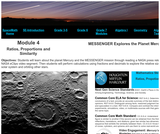Unrestricted Use
Public Domain
Rating

This is a set of four, one-page problems about the size of planets compared to earth. Learners may use ratios to compare planets within our solar system or those outside of our solar system with the earth. Options are presented so that students may learn about the MESSENGER mission to Mercury through a NASA press release or by viewing a NASA eClips video [6 min.]. This activity is part of the Space Math multi-media modules that integrate NASA press releases, NASA archival video, and mathematics problems targeted at specific math standards commonly encountered in middle school.

Subject:
Mathematics
Astronomy
Material Type:
Lesson
Provider:
NASA
Provider Set:
Space Math
11/05/2014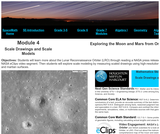Unrestricted Use
Public Domain
Rating

This is a set of three, one-page problems about the scale of objects in images returned by spacecraft. Learners will measure scaled drawings using high-resolution images of the lunar and martian surfaces. Options are presented so that students may learn about the Lunar Reconnaissance Orbiter (LRO) mission through a NASA press release or by viewing a NASA eClips video [4 min.]. This activity is part of the Space Math multi-media modules that integrate NASA press releases, NASA archival video, and mathematics problems targeted at specific math standards commonly encountered in middle school.

Subject:
Mathematics
Astronomy
Material Type:
Lesson
Provider:
NASA
Provider Set:
Space Math
11/05/2014Unrestricted Use
Public Domain
Rating

In this activity book, your students will study five key stages in the lifecycle of a solar storm, from its emergence on the solar surface to its impact upon some aspect of our lives. The book may be used in its entirety to study solar activity and how it directly affects us, or you may use individual activities of your choice as stand-alone mini lessons as an enrichment for math and physical science courses. The student activities emphasize basic cognitive skills and higher-order processes such as plotting data, searching for patterns and correlations, and interpreting the results. By the end of the activity series, students will understand why we need to pay more attention to solar storms.

Subject:
Astronomy
Material Type:
Lesson Plan
Provider:
NASA
Provider Set:
Space Math